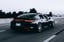# Step 4: Customizing Vector Stores

Customizing the Deep Lake Vector Store

## How to Customize Deep Lake Vector Stores for Images, Multi-Embedding Applications, and More.

Under-the-hood, Deep Lake vector stores use the Deep Lake tabular format, where `Tensors` are conceptually equivalent to columns. A unique feature in Deep Lake is that Tensors can be customized to a variety of use-cases beyond simple embeddings of text.

### Creating vector stores with non-text data

To create a Vector Store for images, we should write a custom embedding function that embeds images from a file using a neural network, since we cannot use OpenAI for embedding images yet.
import os
import torch
from torchvision import transforms, models
from torchvision.models.feature_extraction import create_feature_extractor
from PIL import Image
model = models.resnet18(pretrained=True)
return_nodes = {
"avgpool": "embedding"
}
model = create_feature_extractor(model, return_nodes=return_nodes)
model.eval()
model.to("cpu")
tform = transforms.Compose([
transforms.Resize((224,224)),
transforms.ToTensor(),
transforms.Lambda(lambda x: torch.cat([x, x, x], dim=0) if x.shape == 1 else x),
transforms.Normalize([0.485, 0.456, 0.406], [0.229, 0.224, 0.225]),
])
def embedding_function(images, model = model, transform = tform, batch_size = 4):
"""Creates a list of embeddings based on a list of image filenames. Images are processed in batches."""
if isinstance(images, str):
images = [images]
#Proceess the embeddings in batches, but return everything as a single list
embeddings = []
for i in range(0, len(images), batch_size):
batch = torch.stack([transform(Image.open(item)) for item in images[i:i+batch_size]])
batch = batch.to("cpu")
embeddings+= model(batch)['embedding'][:,:,0,0].cpu().numpy().tolist()
return embeddings
Lets download and unzip 6 example images with common objects and create a list of containing their filenames.
common_objects.zip
485KB
Binary
data_folder = '/Users/istranic/ActiveloopCode/Datasets/common_objects'
image_fns = [os.path.join(data_folder, file) for file in os.listdir(data_folder) if os.path.splitext(file)[-1]=='.jpg']
Earlier in this tutorial, we did not specify any data-structure-related information when initializing the Vector Store, which by default creates a vector store with tensors for `text`, `metadata`, `id (auto-populated)`, and `embedding`.
Here, we create a Vector Store for image similarity search, which should contains tensors for the `image`, its `embedding`, and the `filename` for the image. This can be achieved by specifying custom `tensor_params`.
vector_store_path = '/vector_store_getting_started_images"
vector_store = VectorStore(
path = vector_store_path,
tensor_params = [{'name': 'image', 'htype': 'image', 'sample_compression': 'jpg'},
{'name': 'embedding', 'htype': 'embedding'},
{'name': 'filename', 'htype': 'text'}],
)
We add data to the Vector Store just as if we were adding text data earlier in the Getting Started Guide.
filename = image_fns,
embedding_function = embedding_function,
embedding_data = image_fns)
Let's find the image in the Vector Store that is most similar to the reference image below.reference_image.jpg
43KB
Image
image_path = '/reference_image.jpg'
result = vector_store.search(embedding_data = [image_path], embedding_function = embedding_function)
We can display the result of the most similar image, which shows a picture of a yellow Lamborghini, which is fairly similar to the black Porsche above.
Image.fromarray(result['image'])COMING SOON